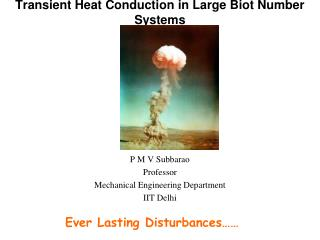DownloadDownload PresentationTransient Heat Conduction in Large Biot Number Systems

# Transient Heat Conduction in Large Biot Number Systems

Télécharger la présentation## Transient Heat Conduction in Large Biot Number Systems

- - - - - - - - - - - - - - - - - - - - - - - - - - - E N D - - - - - - - - - - - - - - - - - - - - - - - - - - -
##### Presentation Transcript

1. Transient Heat Conduction in Large Biot Number Systems P M V Subbarao Professor Mechanical Engineering Department IIT Delhi Ever Lasting Disturbances……

2. Systems with Negligible Surface Resistance • Homeotherm is an organism, such as a mammal or bird, having a body temperature that is constant and largely independent of the temperature of its surroundings.

3. Biot Number of Small Birds Bi Fur Thickness, cm

4. Biot Number of Big Birds Fur Thickness, cm

5. Very Large Characteristic Dimension

6. Very Large Characteristic Dimension The United States detonated an atomic bomb over Nagasaki on August 9, 1945. The bombings of Nagasaki and Hiroshima immediately killed between 100,000 and 200,000 people and the only instances nuclear weapons have been used in war.

7. The semi-infinite solid Governing Differential Equation: Boundary conditions x = 0 :T = Ts As x → ∞ :T → T0 Initial condition t = 0 :T = T0

8. Semi Infinite Walls Boundary conditions x = 0 :-kT/ x=q’’ As x → ∞ :T → T0

9. Constant Heat Flux Boundary Condition q’’ Boundary conditions x = 0 :-kT/ x=h(T∞ -T(0.t)) As x → ∞ :T → T0

10. Notice that there is no natural length-scale in the problem. Indeed, the only variables are T, x, t, and α.

11. Transform the derivatives :

12. Using the conditions at x = 0

13. h

14. The surface heat flux:

15. Constant Surface Heat Flux Surface convection

16. Submit an (handwritten only) Assignment proving the surface convection and Constant surface heat flux problems.Date of submission : 8th Oct 2008.

17. Heisler Parameters • Heisler divided the problem into two parts. • Part 1 : Instantaneous center line temperature. Variables are q0,,L, t, and a. • Part 2 : Spatial temperature distribution for a given center line temperature at any time. Variables are : qcenter,,x,L, and a. • Two different charts were developed. • Three parameters are needed to use each of these charts: • First Chart : • Normalized centerline temperature, • the Fourier Number, • and the Biot Number. • The definition for each parameter are listed below:

18. Mid Plane Temperature of Slab

19. Second Chart : Frozen Time Parameter • Normalized local temperature, • Biot Number. • Spatial Location. • The definition for each parameter are listed below:

20. Temperature Distribution in A Slab

21. Internal Energy Lost by the Slab E0 is the Initial internal energy possessed by the slab by virtue of T0 After a time t, the slab has a temperature distribution, T(x,t): Let Qis the change in Initial internal energy of the slab during time t Define a non-dimensional change in energy: With

22. Change in Internal Energy of A Slab

23. Centre Line Temperature of An Infinite Cylinder

24. Temperature Distribution in An Infinite Cylinder

25. Change in Internal Energy of An Infinite Cylinder

26. Centre Temperature of A Sphere

27. Temperature Distribution in A Sphere

28. Change in Internal Energy of A Sphere

29. Multi-dimensional Transient Conduction Finite Cartesian Bodies: Finite Cylindrical Bodies:

30. Multi-dimensional Conduction • The analysis of multidimensional conduction is simplified by approximating the shapes as a combination of two or more semi-infinite or 1-D geometries. • For example, a short cylinder can be constructed by intersecting a 1-D plate with a 1-D cylinder. • Similarly, a rectangular box can be constructed by intersecting three 1-D plates, perpendicular to each other. • In such cases, the temperature at any location and time within the solid is simply the product of the solutions corresponding to the geometries used to construct the shape. • For example, in a rectangular box, T(x*,y*,z*,t) - the temperature at time t and location x*, y*, z* - is equal to the product of three 1-D solutions: T1(x*,t), T2(y*,t), and T3(z*,t).

31. Transient Conduction in A Finite Cylinder